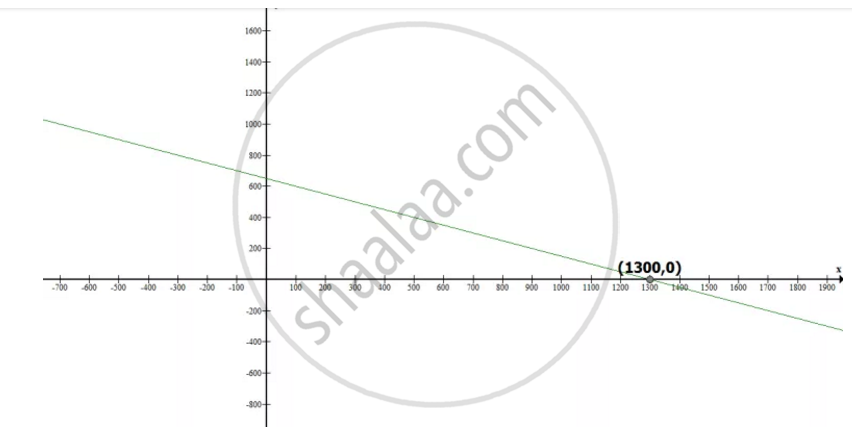# The coach of a cricket team buys 3 bats and 6 balls for Rs 3900. Later, she buys another bat and 3 more balls of the same kind for Rs 1300. Represent this situation algebraically and geometrically. - Mathematics

The coach of a cricket team buys 3 bats and 6 balls for Rs 3900. Later, she buys another bat and 3 more balls of the same kind for Rs 1300. Represent this situation algebraically and geometrically.

#### Solution

Let cost of one bat = Rs x

Cost of one ball = Rs y

3 bats and 6 balls for Rs 3900 So that

3x + 6y = 3900 … (i)

Dividing equation by 3, we get

x + 2y = 1300

Subtracting 2y both side we get

x = 1300 – 2y

Putting y = -1300, 0 and 1300 we get

x = 1300 – 2 (-1300) = 1300 + 2600 = 3900

x = 1300 -2(0) = 1300 - 0 = 1300

x = 1300 – 2(1300) = 1300 – 2600 = - 1300

 x 3900 1300 -1300 y -1300 0 1300

Given that she buys another bat and 2 more balls of the same kind for Rs 1300 So, we get

x + 2y = 1300 … (ii)

Subtracting 2y both side we get

x = 1300 – 2y

Putting y = - 1300, 0 and 1300 we get

x = 1300 – 2 (-1300) = 1300 + 2600 = 3900

x = 1300 – 2 (0) = 1300 - 0 = 1300

x = 1300 – 2(1300) = 1300 – 2600 = -1300

 x 3900 1300 -1300 y -1300 0 1300

Algebraic representation

3+ 6y = 3900 … (i)

+ 2= 1300 … (ii)

Graphical representation,Concept: Pair of Linear Equations in Two Variables
Is there an error in this question or solution?

#### APPEARS IN

NCERT Class 10 Maths
Chapter 3 Pair of Linear Equations in Two Variables
Exercise 3.1 | Q 2 | Page 44